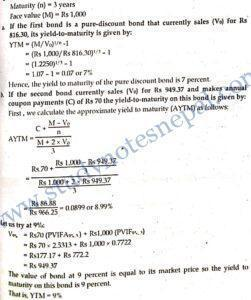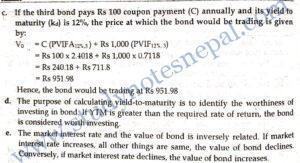###### 1. What do you mean by yield to call?

An yield to call is the annualized rate of return that bondholders will realize if the bond is called at the stated date before the maturity.

###### 2. What does bond’s duration indicate?

Bond’s duration indicates the weighted average number of years that cash flows occur from a bond. It is the measure of interest rate risk of the bond. Higher the bond’s duration higher will be the interest rate risk on the bond.

3. Last year, ABC Company issued 8 percent coupon, 10 years maturity bond that was sold at par value of Rs. 1,000. This year, the market interest rates on similar risk class bonds have increased to 10 percent. What is the value of the bond today?

SOLUTION Given:

Annual coupon (1) = 0.08 x Rs 1,000 = Rs 80

Time to maturity (n) = 10-1=9 years

Maturity value (M)= Rs 1,000

Market interest rate (K) = 10%

Value of the bond today is given by:

Vo =  I x (PVIFA,kd%,n) + Mx (PVIE, kd%,n)

= Rs 80 x 5.7590 + Rs 1,000 × 0.4241

= Rs 460.72 + Rs 424.10

= Rs 884.82

###### 4. A zero coupon bond with Rs 1,000 face value and 3 years to maturity is currently selling at Rs 890. What is its yield to maturity?

SOLUTION

Given:

Face value (M) = Rs 1,000

Time to maturity (n) = 3 years

Selling price of bond (Vo) = Rs 890

The yield to maturity on the bond is given by:

=YTM (M/V)^1/n -1

= 1.0396-1=0.0396 or 3.96%

###### 5. What is the value of a 10 percent perpetual bond if bondholders’’ required rate of return is 12 percent?

SOLUTION

Given:

Coupon rate= 10%

Annual coupon amount (1) = 0.10 x Rs 1,000 = Rs 1004

Required rate of return (ka) = 12%

Thus, value of the perpetual bond is given by:

Vo = I / kd Rs 100 / 0.12

= Rs 833.33

###### 1. What is yield-to-maturity? What is its significance to an investor?

Yield to maturity is the annualized rate of return that an investor can realize if the bond is held until its maturity. This rate of return is based on the certain assumptions. First the bond should be held until the maturity. Second, the bond should pay all promised cash flows. Third, the bond should not be called by the issuer before maturity. And fourth, all intermediate cash flows in terms of coupon interest from bonds are assumed to reinvest at the rate of return equal to YTM.

The basic significance of the yield to maturity is that the investor can use yield to maturity while making investment decision about bond. Specially, the yield to maturity is compared against the required rate of return of bondholders to identify the bonds that are mis-priced. If yield to maturity on the bond is higher than the required rate of return, the bond is said to be undervalued. The investor can buy the undervalued bond because its price is expected to increase until maturity. Conversely, the yield to maturity on the bond is lower than the required rate of return, the bond is said to be overvalued. The investor should sell short the overvalued bond because its price is expected to decline until maturity.

###### 2. Suppose the NMB Bond has 8 percent coupon rate, 10 years until maturity, Rs. 1,000 par value that currently sells for Rs. 1,050.
• What is the current yield on the bond?
• What is its approximate yield to maturity?
• Assume that market interest rate declines to 6 percent in one year. What will be the rate of return if you buy the bond at current selling price today and sell the bond at the end of year ?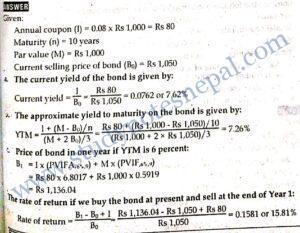###### 3. 2070 Q.No. 8b

Everest Bank Ltd. issued a bond of Rs 10,000 par value with 5 years time to maturity. The coupon rate is 8 percent, paid semi-annually and going market interest rate on similar security is 10 percent. Calculate the duration of the bond and interpret.

SOLUTION

Face value (M) = Rs 10,000

Annual coupon rate (c) = 8%

Maturity period (n) = 5 years

YTM (y) = 10%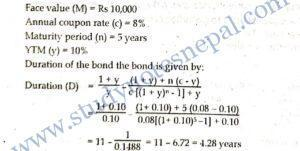Hence, the duration of the bond is 4.28 years. It indicates the weighted average number of years that cash flows occur from the bond. Duration is a better measure of tin.e structure of bonds than years to maturity because duration reflects the amount and timing of every cash flow rather than merely the length of time until the final payment occurs. Moreover, duration is a measure of bond’s interest rate risk.

###### 4. Consider the following information.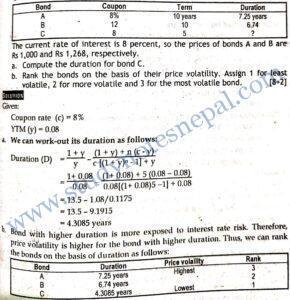###### 5. Consider the following information on a corporate bond:

Face value = Rs 1,000; Annual coupon rate = 10%; Payable semi-annually, maturity period = 3 yrs; YTM = 16%

• Calculate the duration of the bond assuming interest is payable annually.
• Why duration is calculated?

SOLUTION

Given:

Face value (M) = Rs 1,000

Annual coupon rate (c) = 10%

Maturity period (n) = 3 years

YTM (v) = 16%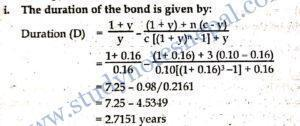The duration of the bond is 2.7151 years. It indicates the weighted average number of years that cash flows occur from the bond. Duration is a better measure of time structure of bonds than years to maturity because duration reflects the amount and timing of every cash flow rather than merely the length of time until the final payment occurs. Moreover, duration is a measure of bond’s interest rate risk. So duration is calculated.

###### 6. Calculate the duration of a bond from the following information:

Face value Rs 1,000

Yield to maturity  15%

Coupon rate (paid annually)  10%

Maturity period of the bond  5 years

SOLUTION Given:

Face value (M) = Rs 1,000

Yield to maturity (y) =15%

Coupon rate (c) = 10%

Maturity period (n) = 5 years

The duration of the bond is given by: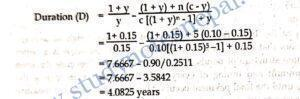The duration of the bond is 4.0825 years. It indicates the w weighted average number of years that cash flows occur from the bond. Duration is a better measure of time structure of bonds than years to maturity because duration reflects the amount and timing of every cash flow rather than merely the length of time until the final payment occurs. Moreover, duration is a measure of bond’s interest rate risk.

###### 7. Find the yield to maturity on a zero coupon bond having face value Rs 1000. Assume that such bond is selling at Rs 880 and has a maturity period 5 years.

SOLUTION

Given:

Face value (M) = Rs 1,000

Selling price (Vd) = Rs 880.

Maturity period (n) = 5 years

The yield to maturity on the zero coupon and is given by: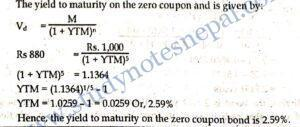###### 8. Consider the following information on a corporate bond: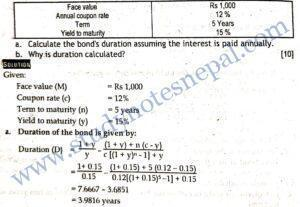The duration of the bond is 3.9816 years. It indicates the weighted average number of years that cash flows occur from the bond. Duration is a better measure of time structure of bonds than years to maturity because duration reflects the amount and timing of every cash flow rather than merely the length of time until the final payment occurs. Moreover, duration is a measure of bond’s interest rate risk. So, duration is calculated.

7. Calculate the value of a bond if its yield to maturity is 14 percent per annum, its coupon is 10 percent per annum, its face value is Rs 1,000, and interest is paid semi-annually. Assume the bond is purchased on an interest payment date and matures in 12 years. Also calculate the value of a bond using the effective semi-annual yield.

SOLUTION

Given:

Yield to maturity (kd) = 14%

Coupon rate (c) = 10%

Face value or Maturity value (M) = Rs 1,000

Interest payment = Semi-annually

Maturity period (n) = 12 years

The value of bond is given by: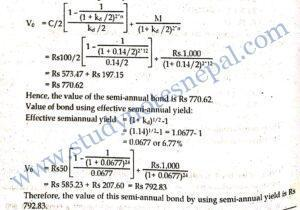###### 1. Grand Bank has three bonds outstanding each with three years to maturity and a face value of Rs 1,000.
1. The first bond is a pure-discount bond that currently sales for Rs 816.30. What is its yield-to-maturity?
2. The second bond currently sales for Rs 949.37 and makes annual coupon payments at a rate of 7% (i.e. it pays Rs 70 in interest each year). The first interest payment is due one year from today. What is this bond’s yield-to-maturity?
3. The third bond pays Rs 100 coupon payment annually. AT what price would the bond be trading if its yield to maturity is 12%?
4. What is the purpose of calculating yield-to-maturity to an investor?
5. Explain the relationship between market interest rate and the value of the bond.

SOLUTION

Given:

Maturity (n) = 3 years

Face value (M)= Rs 1,000